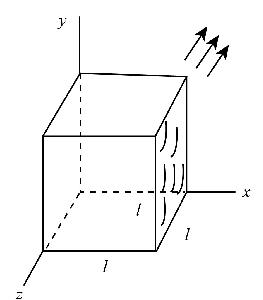# A cube of edge length l = 3.20 cm is positioned. A uniform magnetic field given by \vec{B}= (5.3...

## Question:

A cube of edge length {eq}l = 3.20 cm {/eq} is positioned. A uniform magnetic field given by {eq}\vec{B}= (5.3 \hat{i} + 4.0\hat{j} + 3.0\hat{k} ) T {/eq} exists throughout the region.

(a) Calculate the magnetic flux through the shaded face.

(b) What is the total flux through the six faces?

## Magnetic flux:

The magnetic flux defines as the amount of magnetic field passing through the surface of a conducting coil. Mathematically it is the product of the magnetic field, and the area which is perpendicular to the magnetic field and its standard unit is weber.

Given Data:

• The edge length of a cube is, {eq}l = 3.20\;{\rm{cm}} = 3.20 \times {10^{ - 2}}\;{\rm{m}} {/eq}
• The magnetic field of {eq}\overrightarrow B {/eq} is, {eq}\left( {5.3\widehat i + 4.0\widehat j + 3.0\widehat k} \right){\rm{T}} {/eq}The expression for the magnetic flux is,

{eq}\phi = \overrightarrow {B \cdot } \overrightarrow A {/eq} ........ (1)

Here, {eq}\overrightarrow A {/eq} is the area of the face in the direction of normal to the surface and the unit vector the area is {eq}\widehat i {/eq}

Part (a):

Substitute the value in equation (1)

{eq}\begin{align*} {\phi _1} &= \left( {5.3\widehat i + 4.0\widehat j + 3.0\widehat k} \right) \cdot {\left( {3.20 \times {{10}^{ - 2}}} \right)^2}\widehat i\\ {\phi _1} &= 5.3 \times {\left( {3.20 \times {{10}^{ - 2}}} \right)^2}\\ {\phi _1} &= 5.42 \times {10^{ - 3}}\;{\rm{Wb}} \end{align*} {/eq}

Thus, the magnetic flux through the face is, {eq}5.42 \times {10^{ - 3}}\;{\rm{Wb}} {/eq}.

Part (b):

Substitute the values in equation (1)

{eq}\begin{align*} {\phi _2} &= \left( {5.3\widehat i + 4.0\widehat j + 3.0\widehat k} \right) \cdot {\left( { - 3.20 \times {{10}^{ - 2}}} \right)^2}\widehat i\\ \phi &= - 5.3 \times {\left( {3.20 \times {{10}^{ - 2}}} \right)^2}\\ \phi &= - 5.42 \times {10^{ - 3}}\;{\rm{Wb}} \end{align*} {/eq}

Therefore, the total flux through the faces is calculated as,

{eq}\begin{align*} \phi & = {\phi _1} + {\phi _2}\\ \phi &= 5.42 \times {10^{ - 3}}\; - 5.42 \times {10^{ - 3}}\;\\ \phi &= 0 \end{align*} {/eq}

Thus, the total flux through the faces is zero.